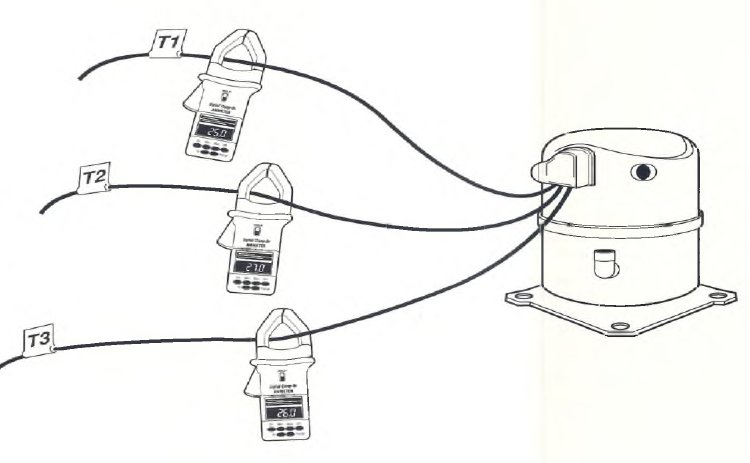﻿ THREE-PHASE COMPRESSOR CURRENT IMBALANCE MEASUREMENT PROCEDURE | HVAC Troubleshooting

## THREE-PHASE COMPRESSOR CURRENT IMBALANCE MEASUREMENT PROCEDURE

StepExpected Result/Action
19. Turn power off. Use the capacitor discharge tool {Figure SP-8-6) to discharge
any capacitors in the unit. Locate the wiring connected to the compressor
motor windings. (Refer to the unit wiring diagram.)
The wiring related to the compressor motor is identified. All high-voltage
capacitors used in the equipment are discharged.
20. Measure the three-phase current as follows:
Check the compressor and/or motor nameplate for full load amps (FLA).
Set up a clamp-on ammeter to measure AC current on the highest range
scale (higher than motor FLA). Place the damp-on ammeter around the
wire connected to ihe T 1 terminal of the compressor as shown in
Figure SP-9-20.
Turn power on, then measure and record the current drawn by the T1 leg.
Repeat the current measurement for the remaining two phases. One at a
time, measure the current flow through the wires connected to the T2 and
T3 terminals of the compressor motor.
Calculate percent of current imbalance for a three-phase system using the
formula below.
% Imbalance = Maximum Current Deviation x , 00
Average Current
Current measured and recorded for the three legs.
The percent current imbalance should be within 10%. (See the example
calculation below.) If current imbalance exceeds 10%, look for an electrical
terminal, contact, etc., that is loose or corroded and is causing a high resistance
in the leg.
Example of % current imbalance calculation
Current measured T1 2 5 amps
Current measured T2 2 7 amps
Current measured T3 26 amps
Average current 7 8 amps + 3 = 2 6 amps
Difference between the average current and the measured current:
T1 - 26 amps - 2 5 amps = 1 amp
T2 = 2 7 amps - 26 amps - 1 amp
T3 = 2 6 amps - 26 amps = 0 amp
% Imbalances Maximum Current Deviation x
Average Current
% Imbalance x 700 = 3 . 8 %
26
Since the imbalance is less than 10%, the current balance between phases is
acceptable.